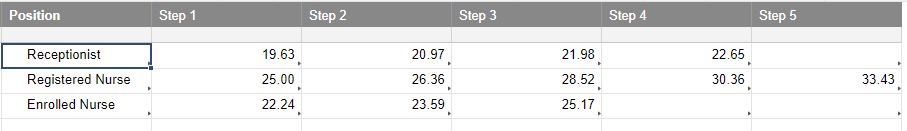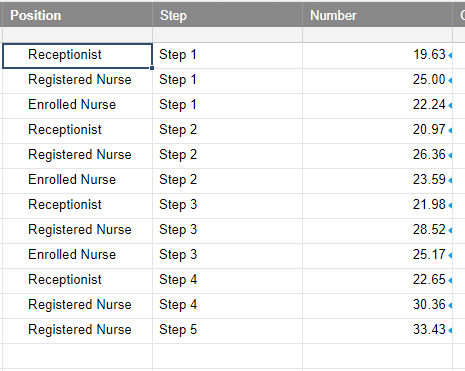# Nested IF , logical operator (AND)

Hi All,

I am trying to build a formula with IF, AND conditions but i am unsuccessful, can any one help me to sort out this please.

Here is the forumula

=IF(AND([Position at Practice]227 = "Receptionist", [Current MECA Step]227 = "Step 1"), "19.63",

IF ([Position at Practice]227 = “Receptionist”, [Current MECA Step]227 = "Step 2"), ”20.97”,

IF ([Position at Practice]227 = “Receptionist”, [Current MECA Step]227 = "Step 3"), ”21.98”,

IF ([Position at Practice]227 = “Receptionist”, [Current MECA Step]227 = "Step 4"), ”22.65”,

IF ([Position at Practice]227 = “Registered Nurse”, [Current MECA Step]227 = "Step 1"), ”25.00”,

IF ([Position at Practice]227 = “Registered Nurse”, [Current MECA Step]227 = "Step 2"), ”26.36”,

IF ([Position at Practice]227 = “Registered Nurse”, [Current MECA Step]227 = "Step 3"), ”28.54”,

IF ([Position at Practice]227 = “Registered Nurse”, [Current MECA Step]227 = "Step 4"), ”30.36”,

IF ([Position at Practice]227 = “Registered Nurse”, [Current MECA Step]227 = "Step 5"), ”33.43”,

IF ([Position at Practice]227 = “Enrolled Nurse”, [Current MECA Step]227 = "Step 1"), ”22.24”,

IF ([Position at Practice]227 = “Enrolled Nurse”, [Current MECA Step]227 = "Step 2"), ”23.59”,

IF ([Position at Practice]227 = “Enrolled Nurse”, [Current MECA Step]227 = "Step 3"), ”25.17”,”0”)

I am getting Unparseable error.

Tags:

• Hi,

Try this.

=IF(AND([Position at Practice]@row = "Receptionist"; [Current MECA Step]@row = "Step 1"); "19.63"; IF(AND([Position at Practice]@row = "Receptionist"; [Current MECA Step]@row = "Step 2"); "20.97"; IF(AND([Position at Practice]@row = "Receptionist"; [Current MECA Step]@row = "Step 3"); "21.98"; IF(AND([Position at Practice]@row = "Receptionist"; [Current MECA Step]@row = "Step 4"); "22.65"; IF(AND([Position at Practice]@row = "Registered Nurse"; [Current MECA Step]@row = "Step 1"); "25.00"; IF(AND([Position at Practice]@row = "Registered Nurse"; [Current MECA Step]@row = "Step 2"); "26.36"; IF(AND([Position at Practice]@row = "Registered Nurse"; [Current MECA Step]@row = "Step 3"); "28.54"; IF(AND([Position at Practice]@row = "Registered Nurse"; [Current MECA Step]@row = "Step 4"); "30.36"; IF(AND([Position at Practice]@row = "Registered Nurse"; [Current MECA Step]@row = "Step 5"); "33.43"; IF(AND([Position at Practice]@row = "Enrolled Nurse"; [Current MECA Step]@row = "Step 1"); "22.24"; IF(AND([Position at Practice]@row = "Enrolled Nurse"; [Current MECA Step]@row = "Step 2"); "23.59"; IF(AND([Position at Practice]@row = "Enrolled Nurse"; [Current MECA Step]@row = "Step 3"); "25.17"; "0"))))))))))))

The same version but with the below changes for your and others convenience.

=IF(AND([Position at Practice]@row = "Receptionist", [Current MECA Step]@row = "Step 1"), "19.63", IF(AND([Position at Practice]@row = "Receptionist", [Current MECA Step]@row = "Step 2"), "20.97", IF(AND([Position at Practice]@row = "Receptionist", [Current MECA Step]@row = "Step 3"), "21.98", IF(AND([Position at Practice]@row = "Receptionist", [Current MECA Step]@row = "Step 4"), "22.65", IF(AND([Position at Practice]@row = "Registered Nurse", [Current MECA Step]@row = "Step 1"), "25.00", IF(AND([Position at Practice]@row = "Registered Nurse", [Current MECA Step]@row = "Step 2"), "26.36", IF(AND([Position at Practice]@row = "Registered Nurse", [Current MECA Step]@row = "Step 3"), "28.54", IF(AND([Position at Practice]@row = "Registered Nurse", [Current MECA Step]@row = "Step 4"), "30.36", IF(AND([Position at Practice]@row = "Registered Nurse", [Current MECA Step]@row = "Step 5"), "33.43", IF(AND([Position at Practice]@row = "Enrolled Nurse", [Current MECA Step]@row = "Step 1"), "22.24", IF(AND([Position at Practice]@row = "Enrolled Nurse", [Current MECA Step]@row = "Step 2"), "23.59", IF(AND([Position at Practice]@row = "Enrolled Nurse", [Current MECA Step]@row = "Step 3"), "25.17", "0"))))))))))))

Depending on your country you’ll need to exchange the comma to a period and the semi-colon to a comma.

I hope this helps you!

Have a fantastic week!

Best,

Andrée Starå

Workflow Consultant @ Get Done Consulting

SMARTSHEET EXPERT CONSULTANT & PARTNER

Andrée Starå | Workflow Consultant / CEO @ WORK BOLD

W: www.workbold.com | E: [email protected] | P: +46 (0) - 72 - 510 99 35

Feel free to contact me about help with Smartsheet, integrations, general workflow advice, or something else entirely.

• I feel like it might be easier to build a table and use an INDEX/MATCH to pull the number. It would allow for much easier updates in the future and provide for less chance of a typo in a super long nested IF statement. The below formula would go in the Number column of the main sheet (second screenshot) and references a table on a separate sheet (first screenshot).

=INDEX({Table Numbers}, MATCH([email protected], {Table Position}, 0), VALUE(RIGHT([email protected])))

{Table Numbers} is a cross sheet reference that encompasses all of the Step columns in the table.

{Table Position} is a cross sheet reference that covers the Position column of the table.thinkspi.com

• Paul,

Nice and I agree, but my experience is that most users prefer the long formulas against the more advanced functions and especially if they are new to Smartsheet.

Great solution regardless!

Have a fantastic weekend!

Best,

Andrée

SMARTSHEET EXPERT CONSULTANT & PARTNER

Andrée Starå | Workflow Consultant / CEO @ WORK BOLD

W: www.workbold.com | E: [email protected] | P: +46 (0) - 72 - 510 99 35

Feel free to contact me about help with Smartsheet, integrations, general workflow advice, or something else entirely.

• True, but sometimes you just gotta go ahead and throw them in the deep end. HAHAHA!!!Edit: We won't throw you in without also tossing in a line for you...

thinkspi.com

•The editSMARTSHEET EXPERT CONSULTANT & PARTNER

Andrée Starå | Workflow Consultant / CEO @ WORK BOLD

W: www.workbold.com | E: [email protected]om | P: +46 (0) - 72 - 510 99 35

Feel free to contact me about help with Smartsheet, integrations, general workflow advice, or something else entirely.